Torsion is tensorial

This article gives the statement, and possibly proof, that a map constructed in a certain way is tensorial
View other such statements

Statement

Let$M$ be a differential manifold and$\nabla$ be a linear connection on$M$ (viz.,$\nabla$ is a connection on the tangent bundle$TM$ of$M$).

Consider the torsion of$\nabla$, namely: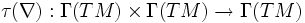$\tau(\nabla): \Gamma(TM) \times \Gamma(TM) \to \Gamma(TM)$

given by: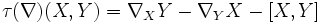$\tau(\nabla)(X,Y) = \nabla_X Y - \nabla_Y X - [X,Y]$

Then,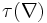$\tau(\nabla)$ is a tensorial map in both coordinates. In other words, the value of$\tau(\nabla)$ at a point$p \in M$ depends only on$\nabla, X(p), Y(p)$ and does not depend on the values of the vectors fields$X,Y$ at points other than$p$.

More explicitly, for any point$p \in M$,$\tau(\nabla)$ defines a bilinear map: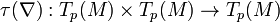$\! \tau(\nabla): T_p(M) \times T_p(M) \to T_p(M)$

Further, since in fact torsion is antisymmetric, this is an alternating bilinear map.

Facts used

Fact no. Name Statement with symbols
1 Any connection is$C^\infty$-linear in its subscript argument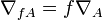$\nabla_{fA} = f\nabla_A$ for any$C^\infty$-function$f$ and vector field$A$.
2 The Leibniz-like axiom that is part of the definition of a connection For a function$f$ and vector fields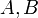$A,B$, and a connection$\nabla$, we have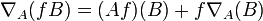$\nabla_A(fB) = (Af)(B) + f\nabla_A(B)$
3 Corollary of Leibniz rule for Lie bracket (in turn follows from Leibniz rule for derivations For a function$f$ and vector fields$X,Y$: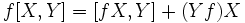$\! f[X,Y] = [fX,Y] + (Yf)X$$\! f[X,Y] = [X,fY] - (Xf)Y$

Proof

To prove tensoriality in a variable, it suffices to show$C^\infty$-linearity in that variable. This is because linearity in$C^\infty$-functions guarantees linearity in a function that is 1 at exactly one point, and zero at others.

The proofs for$X$ and$Y$ are analogous, and rely on manipulation of the Lie bracket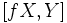$[fX,Y]$ and the property of a connection being$C^\infty$ in the subscript vector.

Tensoriality in the first coordinate

Given: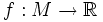$f:M \to \R$ is$C^\infty$-function

To prove: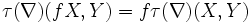$\tau(\nabla)(fX,Y) = f\tau(\nabla)(X,Y)$

Proof: We start out with the left side: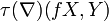$\tau(\nabla)(fX,Y)$

Each step below is obtained from the previous one via some manipulation explained along side.

Step no. Current status of left side Facts/properties used Specific rewrites
1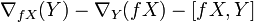$\nabla_{fX}(Y) - \nabla_Y(fX) - [fX,Y]$ Definition of torsion whole thing
2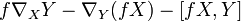$f \nabla_X Y - \nabla_Y(fX) - [fX,Y]$ Fact (1):$C^\infty$-linearity of connection in subscript argument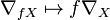$\nabla_{fX} \mapsto f\nabla_X$
3$f \nabla_X Y - (f \nabla_Y X + (Yf)(X)) - [fX,Y]$ Fact (2): The Leibniz-like axiom that's part of the definition of a connection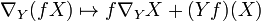$\nabla_Y(fX) \mapsto f\nabla_YX + (Yf)(X)$
4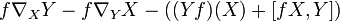$f \nabla_X Y - f \nabla_Y X - ((Yf)(X) + [fX,Y])$ parenthesis rearrangement --
5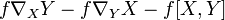$f \nabla_X Y - f \nabla_Y X - f[X,Y]$ Fact (3)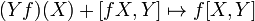$(Yf)(X) + [fX,Y] \mapsto f[X,Y]$
6$f(\nabla_X Y - \nabla_Y X - [X,Y])$ factor out --
7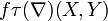$f\tau(\nabla)(X,Y)$ use definition of torsion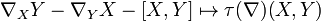$\nabla_X Y - \nabla_Y X - [X,Y] \mapsto \tau(\nabla)(X,Y)$

Tensoriality in the second coordinate

The proof is analogous to that for the first coordinate.

To prove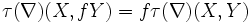$\tau(\nabla)(X,fY) = f \tau(\nabla)(X,Y)$

Proof: This is similar to tensoriality in the first coordinate.